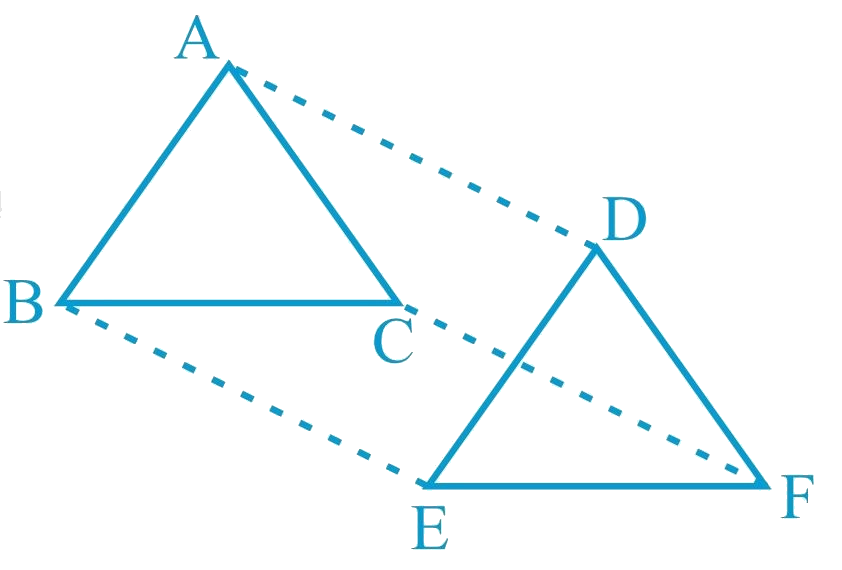# Ex.8.1 Q11 Quadrilaterals Solution - NCERT Maths Class 9

Go back to  'Ex.8.1'

## Question

In $$\Delta ABC \,\rm{and} \,\Delta DEF$$, $$AB \;|| \;DE$$,

$$BC = EF$$ and $$BC\: || \:EF$$. Vertices $$A$$, $$B$$ and $$C$$ are joined to vertices $$D$$, $$E$$ and $$F$$ respectively (see the given figure). Show that(i) Quadrilateral $$ABED$$ is a parallelogram

(ii) Quadrilateral $$BEFC$$ is a parallelogram

(iii) \begin{align} &{ \mathrm{AD} \| \mathrm{CF} \text { and } \mathrm{AD}=\mathrm{CF}} \end{align}

(iv) Quadrilateral $$ACFD$$ is a parallelogram

(v) $$AC = DF$$

(vi) \begin{align} &{ \triangle \mathrm{ABC} \cong \triangle \mathrm{DEF} \text { . }}\end{align}

Video Solution
Ex 8.1 | Question 11

## Text Solution

What is known?

In $$\Delta ABC\text{ }and\text{ }\Delta DEF,$$

$$AB = DE, AB || DE, BC = EF$$ and $$BC || EF.$$

What is unknown?

How we can show that

(i) Quadrilateral $$ABED$$ is a parallelogram

(ii) Quadrilateral $$BEFC$$ is a parallelogram

(iii) $$AD || CF$$ and $$AD = CF$$

(iv) Quadrilateral $$ACFD$$ is a parallelogram

(v) $$AC = DF$$

(vi) $$\Delta ABC\cong \Delta DEF.$$

Reasoning:

We can use the fact that in a quadrilateral if one pair of opposite sides are parallel and equal to each other then it will be a parallelogram and converse is also true. Also by using suitable congruence criterion we can show triangles congruent then we can say corresponding parts of congruent triangles will be equal.

Steps:

(i) It is given that $$AB = DE$$ and $$AB \;|| \;DE$$.

If one pair of opposite sides of a quadrilateral are equal and parallel to each other, then it will be a parallelogram.

Therefore, quadrilateral $$ABED$$ is a

parallelogram.

(ii) Again, $$BC = EF$$ and $$BC\: || \:EF$$

Therefore, quadrilateral $$BCFE$$

is a parallelogram.

(iii) As we had observed that $$ABED$$ and $$BEFC$$ are parallelograms, therefore

$$AD = BE$$ and $$AD \;|| \;BE$$

(Opposite sides of a parallelogram are equal and parallel)

And, $$BE = CF$$ and $$BE\; || \;CF$$

(Opposite sides of a parallelogram are equal and parallel)

$$\therefore$$  $$AD = CF$$ and $$AD \;|| \;CF$$

(iv) As we had observed that one pair of opposite sides ($$AD$$ and $$CF$$) of quadrilateral $$ACFD$$ are equal and parallel to each other, therefore, it is a parallelogram.

(v) As $$ACFD$$ is a parallelogram, therefore, the pair of opposite sides will be equal and parallel to each other.

$$\therefore$$  $$AC\; || \;DF$$ and $$AC = DF$$

(vi) \begin{align} { \triangle \mathrm{ABC} \text { and } \triangle \mathrm{DEF} \text { , }} \end{align}

\begin{align} \mathrm{AB} &=\mathrm{DE}(\text {Given}) \\ \mathrm{BC} &=\mathrm{EF}(\text { Given}) \\ \mathrm{AC} &=\mathrm{DF}\\(\mathrm{ACFD} &\text { is a parallelogram) }\\ \therefore \triangle \mathrm{ABC} &\cong \Delta \mathrm{DEF}\\\text{(By SSS} &\text { congruence rule })\end{align}

Video Solution